# Lecture 32: Bipartite Matchings and Reductions

## Overview

1. Reductions
2. Maximum Bipartite Matchings
3. Reduction to Maximum Flow

## Big Picture, So Far

Efficient algorithms for many problems:

• sorting
• graph problems
• shortest paths
• Eulerian circuits
• minimum spanning trees
• interval scheduling
• sequence alignment
• stable matching

All solved in $O(N^2)$ time ($N =$ size of instance)

## A Question

What algorithmic problems cannot be solved efficiently?

• What do we mean by “efficiently?”

• How could we show that no algorithm can solve a problem efficiently?

• What is an “algorithm,” anyway?

## Last Unit of the Semester

Algorithmic Reality

For many practical problems:

1. no efficient algorithm is known
2. no proof that there isn’t an efficient algorithm

What do we do in this situation?

Reductions & NP Completeness

• focus on relationships between problems
• what does it mean for problem $A$ to be no harder/easier than problem $B$?

## Algorithm Life

Observation. Algorithm design is challenging.

Lifestyle Choice. Avoid designing new algorithms (when you can get away with it).

How?

Idea. Given a new problem $A$, transform it into a problem $B$ you already know how to solve!

Example from homework: Scheduling contractors with bids

## Reductions

Properties of nice transformations:

1. transforming instances of $A$ to instances of $B$ can be done efficiently
2. solution to $B$ instance can be efficiently transformed back to solution for $A$ instance

$1 + 2 =$ reduction from problem $A$ to problem $B$

Coarse notion of efficiency:

• transformations can be done in time $O(N^c)$ for some constant $c$ ($N =$ input size)

In this case reduction is polynomial time reduction

• write $A \leq_P B$ if polynomial time reduction from $A$ to $B$

## Practical Value of Reductions

If $A \leq_P B$, then:

• any efficient solution to $B$ gives an efficient solution to $A$
• an improved algorithm for $B$ may give an improved algorithm for $A$

New Challenge

• Given a problem $A$, solve it by reducing to another problem $B$ that you already have an algorithm for

## Internship Assignments, Again

In a small world…

• Three students: $a, b, c$
• Three internships: $X, Y, Z$

Students/Internships have acceptability criteria (not preferences)

• $a: Y, X$
• $b: Z, X$
• $c: Y, Z$
• $X: a, b$
• $Y: a, c$
• $Z: b, c$

How to match students an internships?

## Viewed as a Graph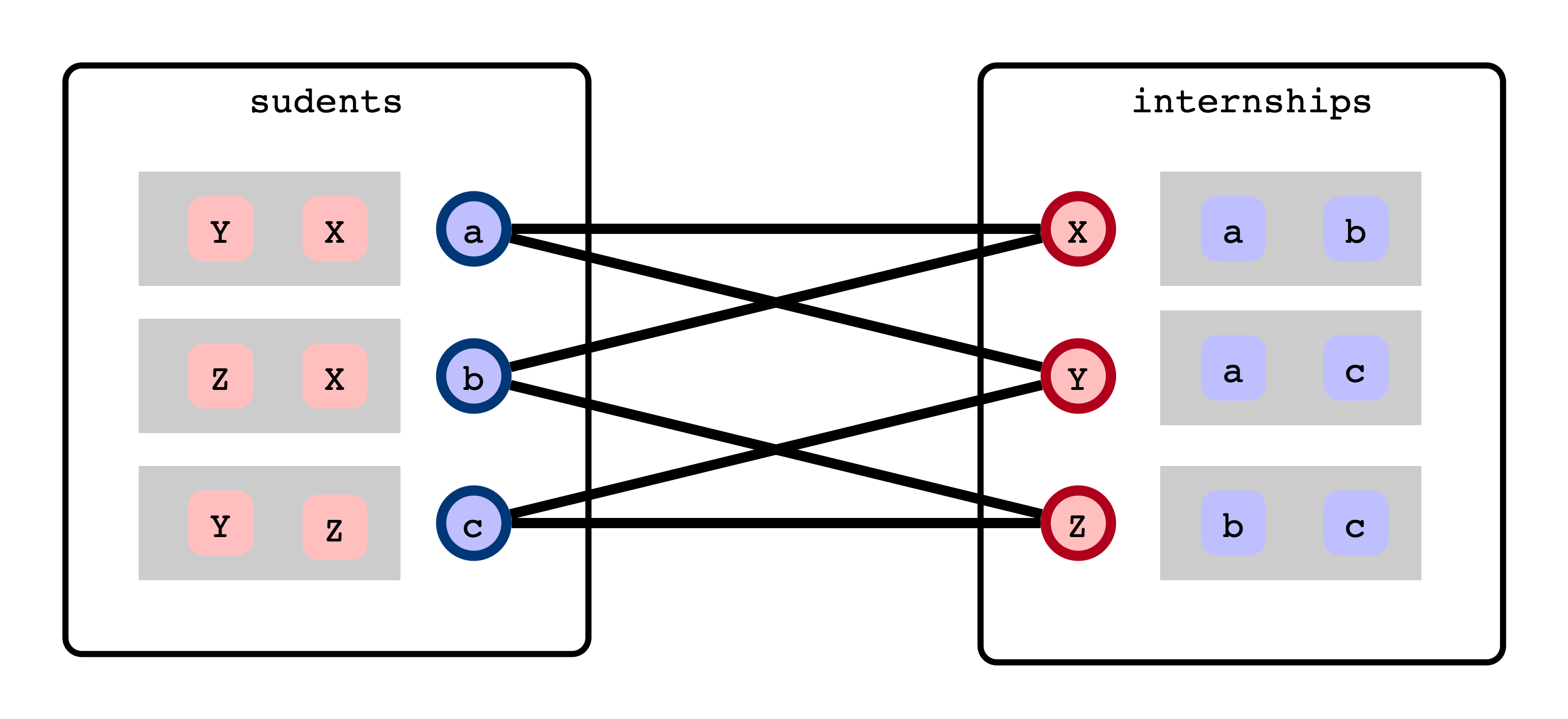## Not Great Matching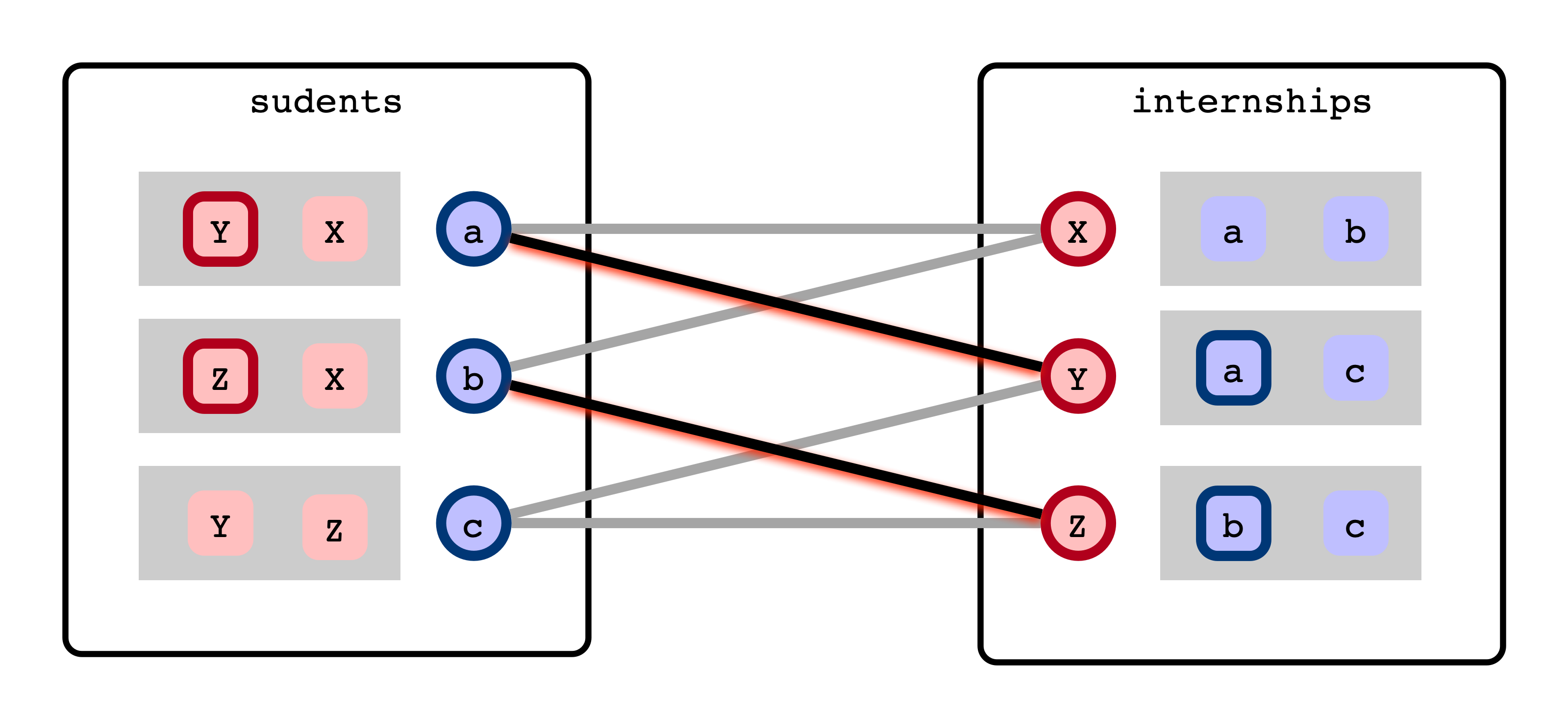## Best Matching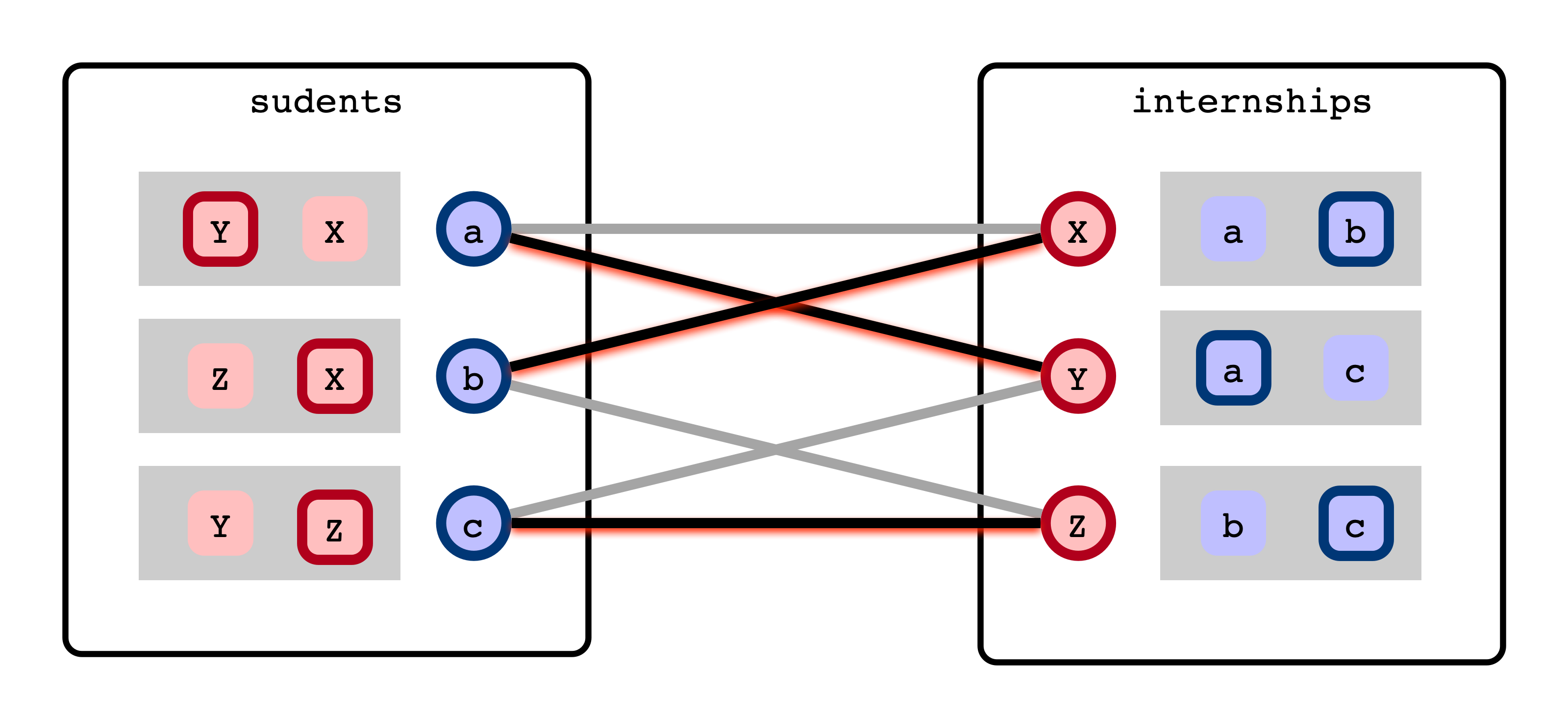## Maximum Bipartite Matching (MBM)

Input:

• bipartite graph $G = (V, E)$
• $V$ partitioned into two disjoint sets, $S, T$
• All edges are pairs $(s, t)$ with $s \in S$, $t \in T$

Output:

• a matching $M = \{(s_1, t_1), (s_2, t_2), \ldots, (s_k, t_k)\}$
• each $(s_i, t_i)$ is an edge in $G$
• each $s \in S$, $t \in T$ appears in at most one pair
• $M$ is a maximum matching: there is no matching of size $\ell > k$

## Simplest Interesting Example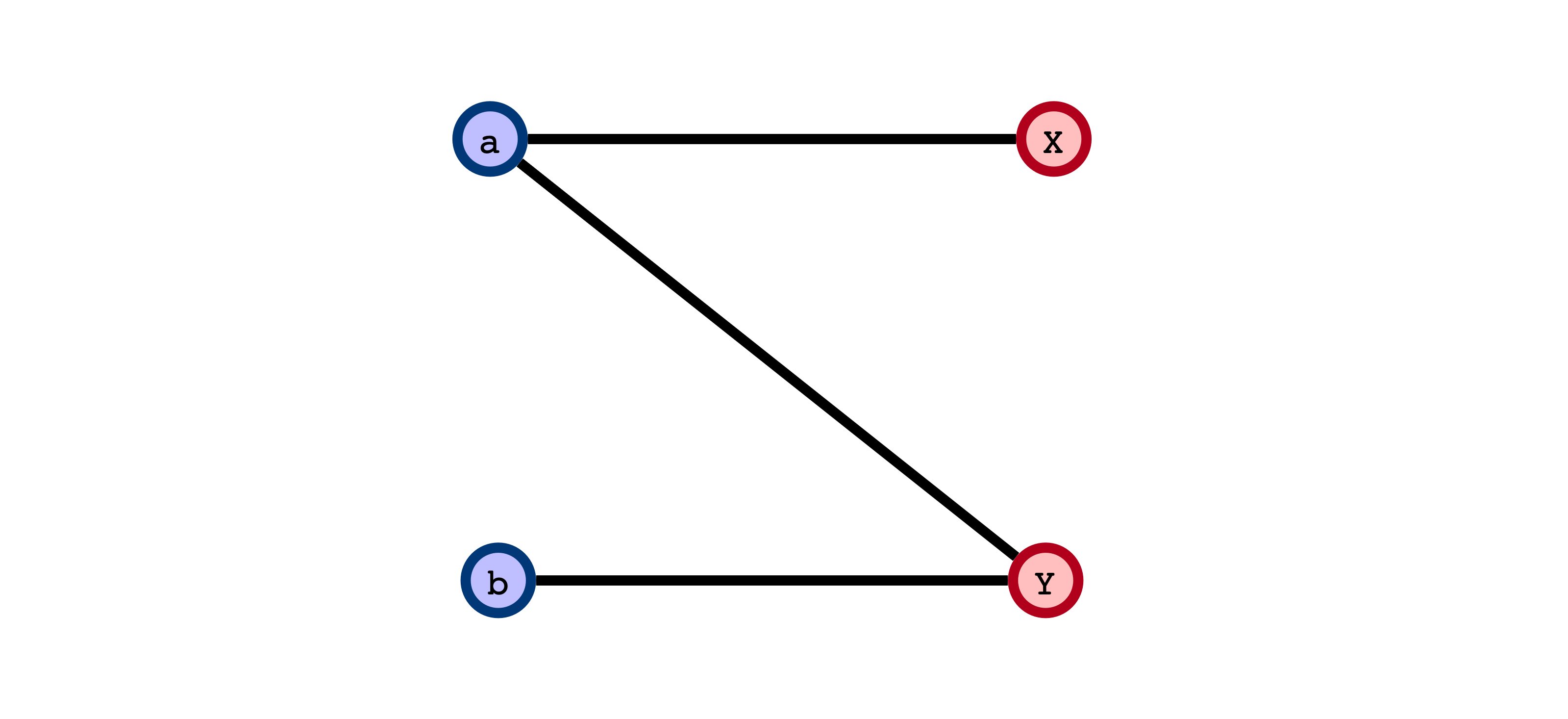Does greedily adding edges to form a matching always result in a maximum matching?

## Greedy Doesn’t WorkIssue: choosing an edge greedily/prematurely may block other edges that could result in a larger matching

• does this sound familiar?

## Adapting to Our New Lifestyle

Don’t design a new algorithm…

Maximum Flow!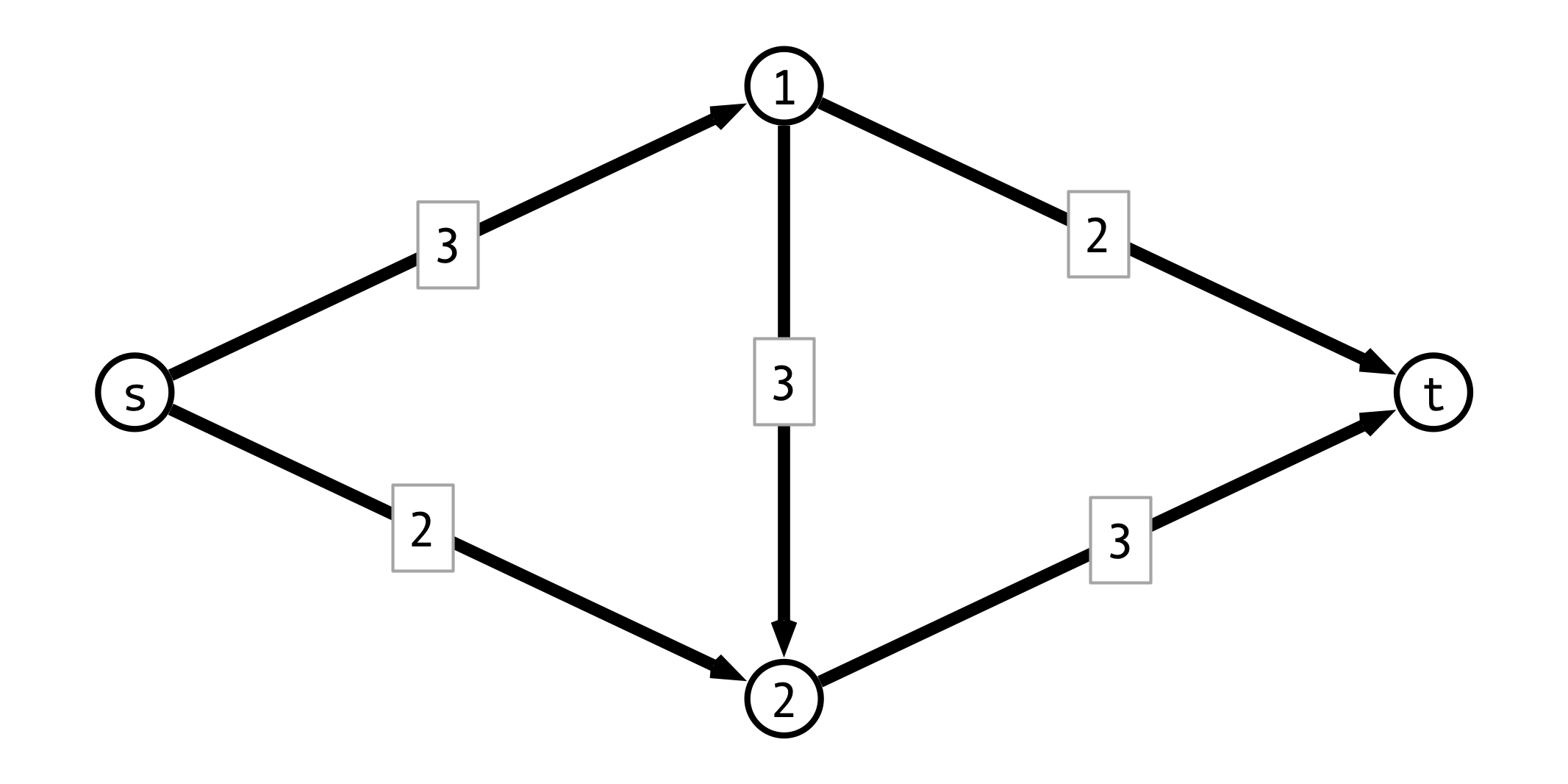## Reduction to Max-Flow

How to turn MBM into a flow problem?## A Max-Flow Instance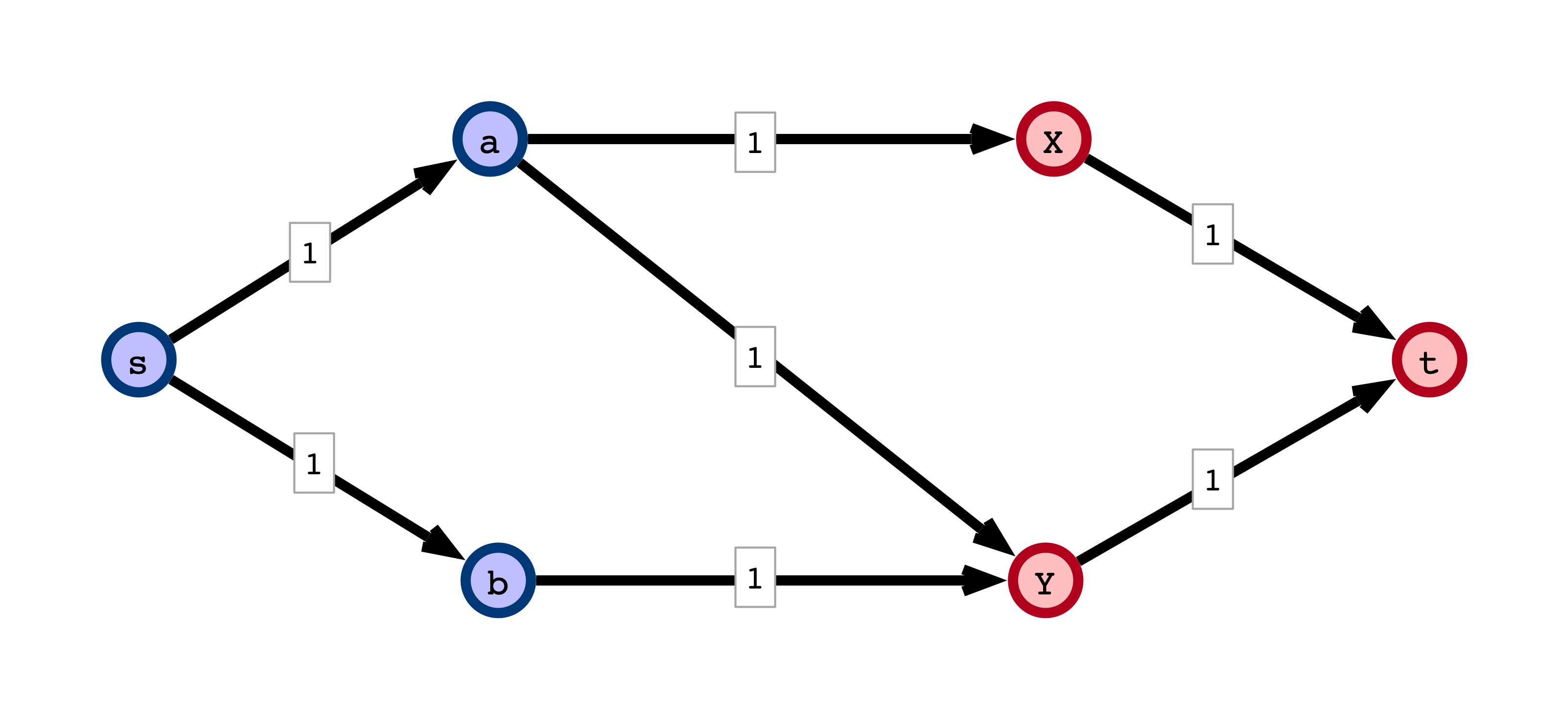## Formalizing the Reduction

Input:

• Bipartite graph $G = (V, E)$ with $V = S \cup T$

Output:

• Directed graph $G’ = (V’, E’)$
• $V’ = V \cup \{s, t\}$
• For $E’$:
• direct all edges $(s_i, t_j) \in E$ from $S$ to $T$
• add edges $(s, s_i)$ for all $s_i \in S$
• add edges $(t_j, t)$ for all $t_j \in T$
• all capacities are $1$## II Form Max Flow Instance## III Solve Max Flow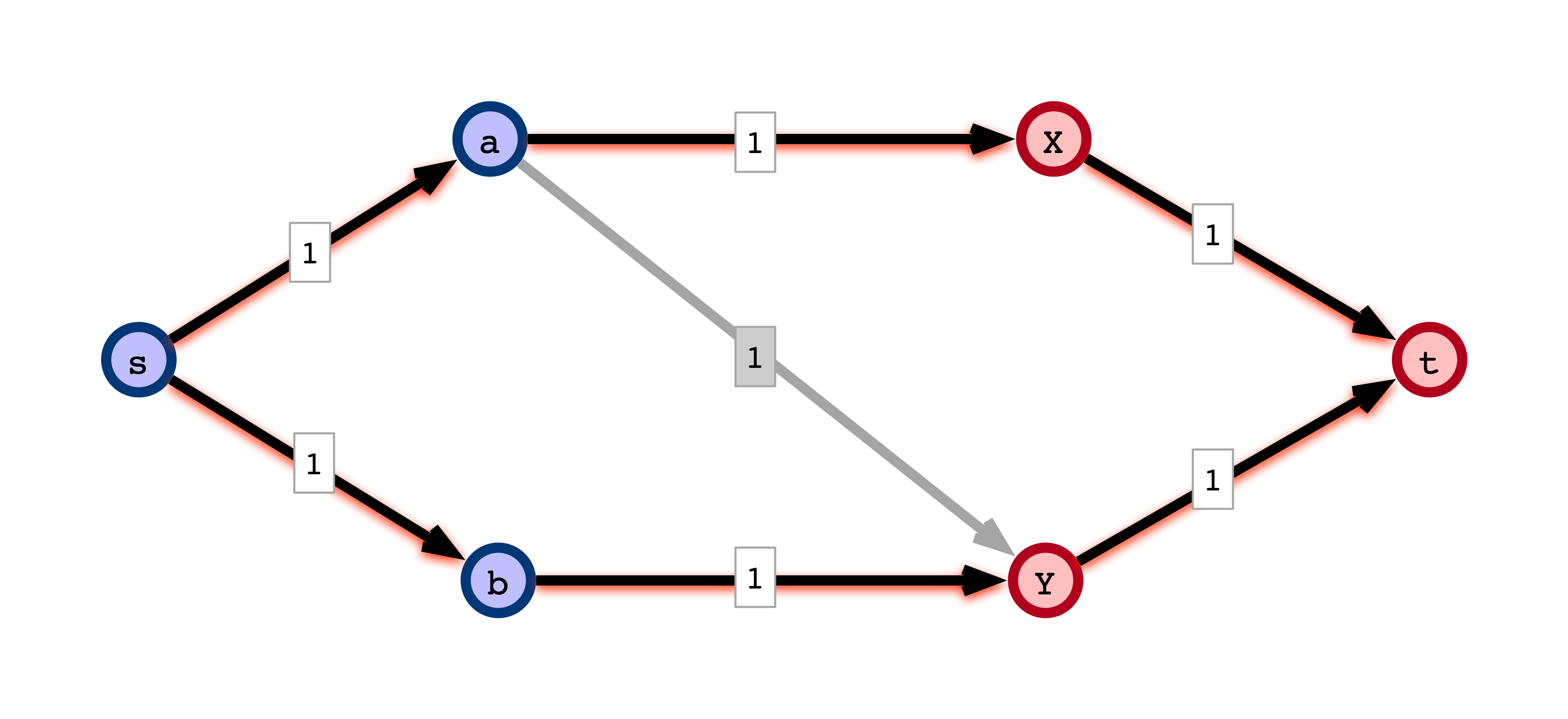## IV Form Matching from Flow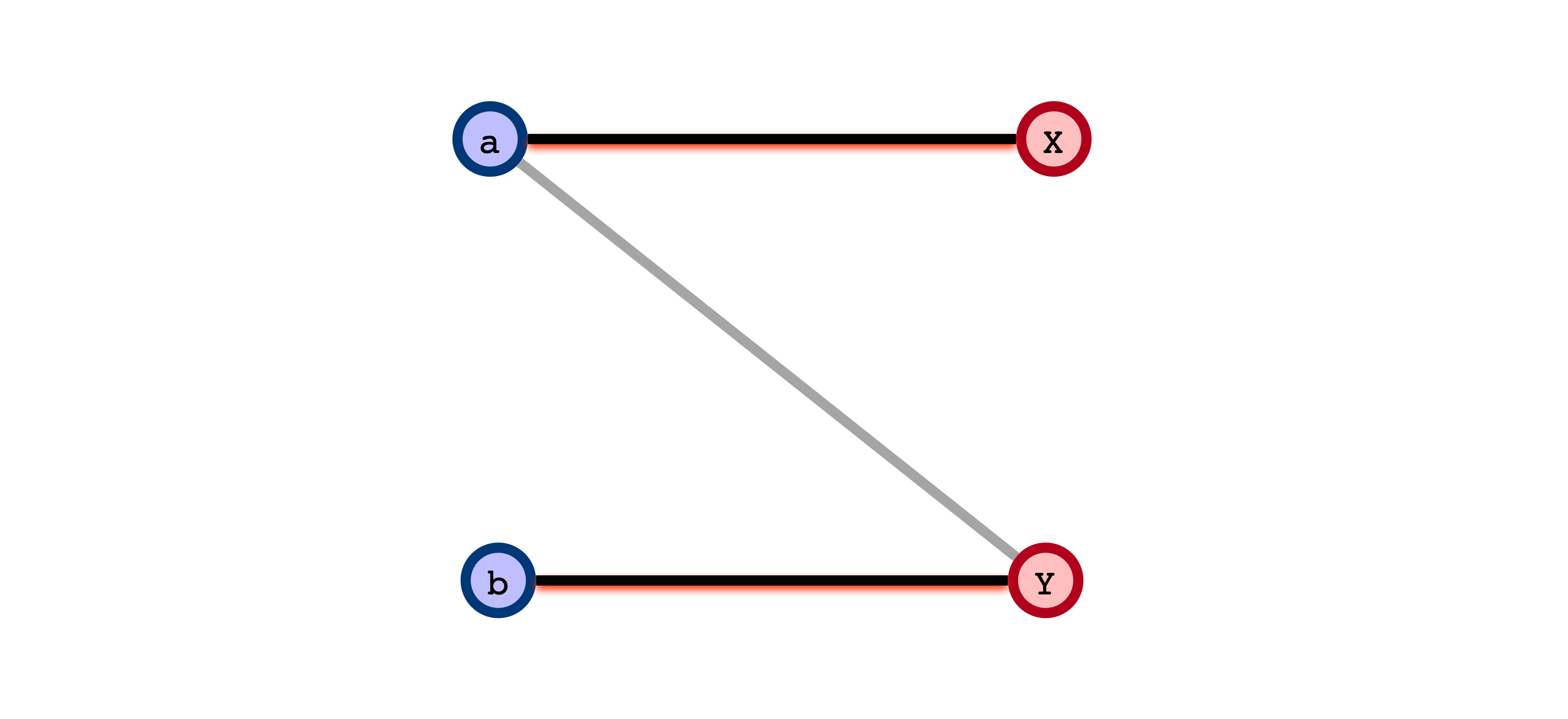## A Technicality

Assume all flows are integral flows:

• amount of flow across each edge is an integerNote. Ford-Fulkerson always gives an integral flow.

## Claim 1

If $G$ has a matching of size $k$, then $G’$ admits a flow $f$ of value $k$.## Claim 2

If $G’$ admits an integral flow of value $k$, then $G$ has a matching of size $k$.## Implications

1. Value of the maximum flow in $G’$ equals the size maximum matching in $G$.
2. Given a maximum (integral) flow in $G’$, we can find a maximum matching in $G$

## Conclusions

• Found a reduction from Maximum Bipartite Matching to Max Flow
• Any (integral) Max Flow algorithm can be used to solve MBM
• any improvement in Max Flow algorithms will yield a corresponding improvement in MBM solution
• We showed MBM $\leq_P$ Max Flow
• MBM is “no harder” than Max Flow
• Max Flow is “no easier” than MBM

## Next Time

Another view of $\leq_P$:

• suppose $A$ is a “hard” problem
• we show $A \leq_P B$
• then we’ve established that $B$ is no easier than $A$
• any efficient solution to $B$ would imply an efficient solution to $A$